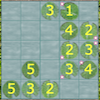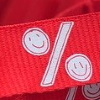# Resources tagged with: Interactivities

Filter by: Content type:
Age range:
Challenge level:

### There are 94 results

Broad Topics > Physical and Digital Manipulatives > Interactivities### Excel Interactive Resource: Fraction Multiplication

##### Age 11 to 16Challenge Level

Use Excel to explore multiplication of fractions.### Sliding Puzzle

##### Age 11 to 16Challenge Level

The aim of the game is to slide the green square from the top right hand corner to the bottom left hand corner in the least number of moves.### Cogs

##### Age 11 to 14Challenge Level

A and B are two interlocking cogwheels having p teeth and q teeth respectively. One tooth on B is painted red. Find the values of p and q for which the red tooth on B contacts every gap on the. . . .### Excel Interactive Resource: Number Grid Functions

##### Age 11 to 16Challenge Level

Use Excel to investigate the effect of translations around a number grid.### Excel Interactive Resource: the up and Down Game

##### Age 11 to 16Challenge Level

Use an interactive Excel spreadsheet to explore number in this exciting game!### Excel Interactive Resource: Equivalent Fraction Bars

##### Age 11 to 16Challenge Level

A simple file for the Interactive whiteboard or PC screen, demonstrating equivalent fractions.### Excel Interactive Resource: Long Multiplication

##### Age 11 to 16Challenge Level

Use an Excel spreadsheet to explore long multiplication.### The Triangle Game

##### Age 11 to 16Challenge Level

Can you discover whether this is a fair game?### Excel Interactive Resource: Multiples Chain

##### Age 11 to 16Challenge Level

Use an interactive Excel spreadsheet to investigate factors and multiples.### Excel Interactive Resource: Fraction Addition & Fraction Subtraction

##### Age 11 to 16Challenge Level

Use Excel to practise adding and subtracting fractions.### Excel Interactive Resource: Haitch

##### Age 11 to 16Challenge Level

An Excel spreadsheet with an investigation.### Jam

##### Age 14 to 16Challenge Level

To avoid losing think of another very well known game where the patterns of play are similar.### Got It

##### Age 7 to 14Challenge Level

A game for two people, or play online. Given a target number, say 23, and a range of numbers to choose from, say 1-4, players take it in turns to add to the running total to hit their target.### Have You Got It?

##### Age 11 to 14Challenge Level

Can you explain the strategy for winning this game with any target?### Excel Interactive Resource: Interactive Division

##### Age 11 to 16Challenge Level

Use an Excel to investigate division. Explore the relationships between the process elements using an interactive spreadsheet.### Cushion Ball Interactivity

##### Age 14 to 16Challenge Level

The interactive diagram has two labelled points, A and B. It is designed to be used with the problem "Cushion Ball"### Colour in the Square

##### Age 7 to 16Challenge Level

Can you put the 25 coloured tiles into the 5 x 5 square so that no column, no row and no diagonal line have tiles of the same colour in them?### Disappearing Square

##### Age 11 to 14Challenge Level

Do you know how to find the area of a triangle? You can count the squares. What happens if we turn the triangle on end? Press the button and see. Try counting the number of units in the triangle now. . . .### Treasure Hunt

##### Age 7 to 14Challenge Level

Can you find a reliable strategy for choosing coordinates that will locate the treasure in the minimum number of guesses?### Partitioning Revisited

##### Age 11 to 14Challenge Level

We can show that (x + 1)² = x² + 2x + 1 by considering the area of an (x + 1) by (x + 1) square. Show in a similar way that (x + 2)² = x² + 4x + 4### Changing Places

##### Age 14 to 16Challenge Level

Place a red counter in the top left corner of a 4x4 array, which is covered by 14 other smaller counters, leaving a gap in the bottom right hand corner (HOME). What is the smallest number of moves. . . .### Picturing Triangular Numbers

##### Age 11 to 14Challenge Level

Triangular numbers can be represented by a triangular array of squares. What do you notice about the sum of identical triangle numbers?### Square It

##### Age 11 to 16Challenge Level

Players take it in turns to choose a dot on the grid. The winner is the first to have four dots that can be joined to form a square.### Number Pyramids

##### Age 11 to 14Challenge Level

Try entering different sets of numbers in the number pyramids. How does the total at the top change?### Interactive Number Patterns

##### Age 14 to 16Challenge Level

How good are you at finding the formula for a number pattern ?### Latin Lilies

##### Age 7 to 14Challenge Level

In this game you are challenged to gain more columns of lily pads than your opponent.### Estimating Angles

##### Age 7 to 14Challenge Level

How good are you at estimating angles?### More Number Pyramids

##### Age 11 to 14Challenge Level

When number pyramids have a sequence on the bottom layer, some interesting patterns emerge...### Drips

##### Age 7 to 14Challenge Level

An animation that helps you understand the game of Nim.### Stars

##### Age 11 to 14Challenge Level

Can you work out what step size to take to ensure you visit all the dots on the circle?### Fractions and Percentages Card Game

##### Age 11 to 16Challenge Level

Can you find the pairs that represent the same amount of money?### Proof Sorter - Quadratic Equation

##### Age 14 to 18Challenge Level

This is an interactivity in which you have to sort the steps in the completion of the square into the correct order to prove the formula for the solutions of quadratic equations.### Guesswork

##### Age 14 to 16Challenge Level

Ask a friend to choose a number between 1 and 63. By identifying which of the six cards contains the number they are thinking of it is easy to tell them what the number is.##### Age 14 to 16Challenge Level

A counter is placed in the bottom right hand corner of a grid. You toss a coin and move the star according to the following rules: ... What is the probability that you end up in the top left-hand. . . .### Countdown Fractions

##### Age 11 to 16Challenge Level

Here is a chance to play a fractions version of the classic Countdown Game.### Factor Lines

##### Age 7 to 14Challenge Level

Arrange the four number cards on the grid, according to the rules, to make a diagonal, vertical or horizontal line.### Last Biscuit

##### Age 11 to 18Challenge Level

Can you find a strategy that ensures you get to take the last biscuit in this game?### Factors and Multiples Game

##### Age 7 to 16Challenge Level

A game in which players take it in turns to choose a number. Can you block your opponent?### A Tilted Square

##### Age 14 to 16Challenge Level

The opposite vertices of a square have coordinates (a,b) and (c,d). What are the coordinates of the other vertices?### Spot the Card

##### Age 14 to 16Challenge Level

It is possible to identify a particular card out of a pack of 15 with the use of some mathematical reasoning. What is this reasoning and can it be applied to other numbers of cards?### Inside Out

##### Age 14 to 16Challenge Level

There are 27 small cubes in a 3 x 3 x 3 cube, 54 faces being visible at any one time. Is it possible to reorganise these cubes so that by dipping the large cube into a pot of paint three times you. . . .### Tilting Triangles

##### Age 14 to 16Challenge Level

A right-angled isosceles triangle is rotated about the centre point of a square. What can you say about the area of the part of the square covered by the triangle as it rotates?### Magic W

##### Age 14 to 16Challenge Level

Find all the ways of placing the numbers 1 to 9 on a W shape, with 3 numbers on each leg, so that each set of 3 numbers has the same total.### Polycircles

##### Age 14 to 16Challenge Level

Show that for any triangle it is always possible to construct 3 touching circles with centres at the vertices. Is it possible to construct touching circles centred at the vertices of any polygon?### Olympic Magic

##### Age 14 to 16Challenge Level

in how many ways can you place the numbers 1, 2, 3 … 9 in the nine regions of the Olympic Emblem (5 overlapping circles) so that the amount in each ring is the same?### Instant Insanity

##### Age 11 to 18Challenge Level

Given the nets of 4 cubes with the faces coloured in 4 colours, build a tower so that on each vertical wall no colour is repeated, that is all 4 colours appear.### Factors and Multiples - Secondary Resources

##### Age 11 to 16Challenge Level

A collection of resources to support work on Factors and Multiples at Secondary level.### Just Rolling Round

##### Age 14 to 16Challenge Level

P is a point on the circumference of a circle radius r which rolls, without slipping, inside a circle of radius 2r. What is the locus of P?### Isosceles Triangles

##### Age 11 to 14Challenge Level

Draw some isosceles triangles with an area of $9$cm$^2$ and a vertex at (20,20). If all the vertices must have whole number coordinates, how many is it possible to draw?### Square Coordinates

##### Age 11 to 14Challenge Level

A tilted square is a square with no horizontal sides. Can you devise a general instruction for the construction of a square when you are given just one of its sides?